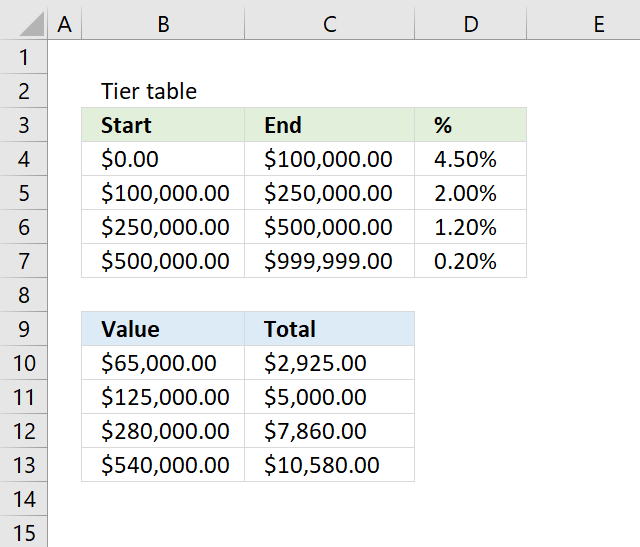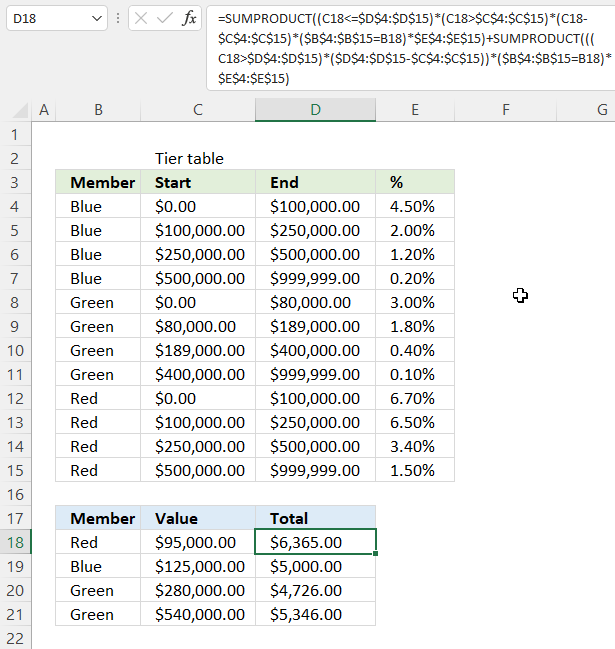Author: Oscar Cronquist Article last updated on November 04, 2022The image above demonstrates a formula that calculates tiered values based on a tier table and returns a total. This kind of formula is often used to calculate commissions, bonuses, pricing, fees or charges, discounts, volume pricing, volume rebate, and performance incentives.

The table shows the different thresholds or levels that a specific percentage applies to, if an amount is larger than the first level then multiple calculations are necessary in order to calculate the total. The formula takes care of these additional calculations as well, no need for helper columns.

I have written an article about how to Use price ranges to determine discounts, it also explains how to calculate linear discounts.

## 1. How to do tiered calculations in one formulaFormula in cell C10:

=SUMPRODUCT((B10<=\$C\$4:\$C\$7)* (B10>\$B\$4:\$B\$7)* (B10- \$B\$4:\$B\$7)* \$D\$4:\$D\$7)+ SUMPRODUCT(((B10>\$C\$4:\$C\$7)* (\$C\$4:\$C\$7- \$B\$4:\$B\$7))* \$D\$4:\$D\$7)

There are four different values B10:B13 in order to demonstrate how the total changes based on the percentage and levels used, I will explain below how each value is calculated.

### 1.1 Explaining calculations

#### 1.1.1 Example 1 - \$65 000If \$65 000 is used then the formula returns \$2 925, here is how that number is calculated: 65 000 * 4.5% = 2 925

The amount is in cell B10 and the calculation is in cell C10, see the image above.

 Amount Percentage Result 65 000 4.5% 2 925 Total 2 925

#### 1.1.2 Example 2 - \$125 000\$125 000 returns \$5 000. 100 000 * 4.5% = 4 500. 25 000 * 2% = 500. 4 500 + 500 = 5 000.

The amount is in cell B11 and the calculation is in cell C11, see the image above.

 Amount Percentage Result 100 000 4.5% 4 500 25 000 2% 500 Total 5 000

#### 1.1.3 Example 3 - \$280 000\$280 000 returns \$7 860. 100 000 * 4.5% = 4 500. 150 000 * 2% = 3 000. 30 000 *  1.2% = 360. 4 500 + 3 000 + 360 = 7 860

The amount is in cell B12 and the calculation is in cell C12, see the image above.

 Amount Percentage Result 100 000 4.5% 4 500 150 000 2% 3 000 30 000 1.2% 360 Total 7 860

#### 1.1.4 Example 4 - \$540 000\$540 000 returns \$10 580. 100 000 * 4.5% = 4 500. 150 000 * 2% = 3 000. 250 000 *  1.2% = 3 000. 40 000 * 0.2% = 80. 4 500 + 3 000 + 3 000 + 80 = 10 580.

The amount is in cell B13 and the calculation is in cell C13, see the image above.

 Amount Percentage Result 100 000 4.5% 4 500 150 000 2% 3 000 250 000 1.2% 3 000 40 000 0.2% 80 Total 10 580

### 1.2 Explaining formula in cell C10

The formula contains two SUMPRODUCT functions, the first one calculates the result based on the amount that is above a specific level and the corresponding percentage.

The second SUMPRODUCT function calculates tiered values based on the amounts up to the reached level and their corresponding percentages. The formula then adds those numbers and returns the total.

Recommended article: How to return a value if lookup value is in a range

#### Step 1 - First SUMPRODUCT function

The first two logical expressions determine which tier the amount in cell B10 reaches.

(B10<=\$C\$4:\$C\$7)* (B10>\$B\$4:\$B\$7)

becomes

(65000<={100000; 250000; 500000; 999999})*(65000>{0; 100000; 250000; 500000})

becomes

({TRUE; TRUE; TRUE; TRUE})*({TRUE; FALSE; FALSE; FALSE})

and returns {1; 0; 0; 0}.

#### Step 2 - Subtract value with tier levels

The parentheses let you control the order of operation, we want to subtract before we multiply the arrays in order to get the correct result we are looking for.

(B10- \$B\$4:\$B\$7)

becomes

(65000 - {0; 100000; 250000; 500000})

and returns {65000; -35000; -185000; -435000}.

#### Step 3 - Multiply arrays

(B10<\$C\$4:\$C\$7)*(B10>\$B\$4:\$B\$7)*(B10- \$B\$4:\$B\$7)* \$D\$4:\$D\$7

becomes

{1; 0; 0; 0}*{65000; -35000; -185000; -435000}*{0.045;0.02;0.012;0.002}

and returns {2925; 0; 0; 0}.

#### Step 4 - Add values

The SUMPRODUCT function calculates the product of corresponding values and then returns the sum of each multiplication.

SUMPRODUCT((B10<\$C\$4:\$C\$7)* (B10>\$B\$4:\$B\$7)* (B10- \$B\$4:\$B\$7)* \$D\$4:\$D\$7)

becomes

SUMPRODUCT({2925;0;0;0})

and returns 2925.

#### Step 5 - Second SUMPRODUCT function

((B10>\$C\$4:\$C\$7)* (\$C\$4:\$C\$7- \$B\$4:\$B\$7))* \$D\$4:\$D\$7

becomes

(65000>{100000;250000;500000;999999})*({100000;150000;250000;499999})*{0.045;0.02;0.012;0.002}

and returns {0;0;0;0}.

#### Step 6 - Add values in array

SUMPRODUCT(((B10>\$C\$4:\$C\$7)* (\$C\$4:\$C\$7- \$B\$4:\$B\$7))* \$D\$4:\$D\$7)

becomes

SUMPRODUCT({0;0;0;0})

and returns 0.

#### Step 7 - Add numbers

SUMPRODUCT((B10<=\$C\$4:\$C\$7)* (B10>\$B\$4:\$B\$7)* (B10- \$B\$4:\$B\$7)* \$D\$4:\$D\$7)+ SUMPRODUCT(((B10>\$C\$4:\$C\$7)* (\$C\$4:\$C\$7- \$B\$4:\$B\$7))* \$D\$4:\$D\$7)

becomes

2925 + 0

and returns 2925 in cell C10.

### 1.3 Final thoughts

The formula can be simplified to:

=SUMPRODUCT(\$D\$4:\$D\$7* ((B10<=\$C\$4:\$C\$7)* (B10>\$B\$4:\$B\$7)* (B10-\$B\$4:\$B\$7)+ ((B10>\$C\$4:\$C\$7)* (\$C\$4:\$C\$7-\$B\$4:\$B\$7))))

but that formula is harder for me to explain.

Excel 365 users can use this smaller dynamic array formula:

=LET(v,\$B\$4:\$B\$7,x,\$C\$4:\$C\$7,y,B10,SUMPRODUCT(\$D\$4:\$D\$7*((y<=x)*(y>v)*(y-v)+((y>x)*(x-v)))))

## 2. How to do tiered calculations in one formula based on a conditionThis example demonstrates a formula that uses multiple tier tables to calculate a total, a condition determines which table to use.

Formula in cell D18:

=SUMPRODUCT((C18<=\$D\$4:\$D\$15)*(C18>\$C\$4:\$C\$15)*(C18-\$C\$4:\$C\$15)*(\$B\$4:\$B\$15=B18)*\$E\$4:\$E\$15)+SUMPRODUCT(((C18>\$D\$4:\$D\$15)*(\$D\$4:\$D\$15-\$C\$4:\$C\$15))*(\$B\$4:\$B\$15=B18)*\$E\$4:\$E\$15)

The following formula works only in Excel 365, however, it does the same thing as the formula above but is much shorter and easier to adjust if your tier tables are larger or smaller.

Excel 365 dynamic array formula in cell D18:

=LET(z,(\$B\$4:\$B\$15=B18), v, \$C\$4:\$C\$15, x, \$D\$4:\$D\$15, y, C18,SUMPRODUCT(\$E\$4:\$E\$15*((y<=x)*(y>v)*(y-v)*z+((y>x)*(x-v)))*z))